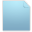###Porting from version 2.0 to 3.1.1

04 Mar 2016 12:44 #1

Hi,

I'm working on porting my chai3d application made in 2.0.0 to 3.1.1. I see bunch of modification on already existing classes. Great if someone can help me to come over some basic issues.
1. How can we access each component of vector in chai3d 3.1.1?. For ex. in 2.0.0 we can simply call

`cVector3d pos; double val = pos1.x`
2. What exactly need to be done in order to display a string?. I did something like this in 2.0.0
`labels[deviceID]->m_string = strLabel;`
but I see in 3.1.1 there is 'm_text'.

Very helpful if someone can give a suggestion on that.
Thank you!

04 Mar 2016 23:23 #2

Here is some code to access vector components using x,y and z references:

```    cVector3d myVector;

// assign values
myVector.x(1.0);
myVector.y(2.0);
myVector.z(3.0);

// retrieve values
double x = myVector.x();
double y = myVector.y();
double z = myVector.z();```

Another way to access a vector's components is by using indices:
```    // assign values
myVector(0) = 1.0;
myVector(1) = 2.0;
myVector(2) = 3.0;

// retrieve values
double x = myVector(0);
double y = myVector(1);
double z = myVector(2);```

Assigning a string to a label can be performed with the following code:
```    string myString = "hello world";
myLabel->setText(myString);```

06 Mar 2016 20:28 #3

Thank you very much!

07 Mar 2016 11:19 #4

Hi,

It would be very helpful if you can suggest a solution for this,

I've made a very basic haptic application using chai3d 2.0.0, now I'm porting everything into 3.1.0. But still getting some issues, not sure where I'm wrong but I suspect it's somewhere in 'updatehaptics'.

This is how I manged to do few manipulations in updatehaptics:

``` hapticDevice->getPosition(newPosition1);
hapticDevice->getPosition(newPosition2);
// read orientation of haptic devices 1, 2

hapticDevice->getRotation(newRotation1);
hapticDevice->getRotation(newRotation2);

// read linear velocity from devices 1,2
hapticDevice->getLinearVelocity(linearVelocity1);
hapticDevice->getLinearVelocity(linearVelocity2);
linearVelocity1.x(0.0);
linearVelocity2.x(0.0);

// update position and orientation of cursors
cursors->setLocalPos(newPosition1);
cursors->setLocalPos(newPosition2);
cursors->setLocalRot(newRotation1);
cursors->setLocalRot(newRotation2);

startPosition1=starts->getLocalPos();
startPosition2=starts->getLocalPos();

targetPosition1=targets->getLocalPos();
targetPosition2=targets->getLocalPos();

viaPosition1 = via->getLocalPos();
viaPosition2 = via->getLocalPos();

//distance from an invisible via point

viadist1 =  (newPosition1.y() - viaPosition1.y())*(newPosition1.y() - viaPosition1.y())+
(newPosition1.z() - viaPosition1.z())*(newPosition1.z() - viaPosition1.z());

viadist2 =  (newPosition2.y() - viaPosition2.y())*(newPosition2.y() - viaPosition2.y())+
(newPosition2.z() - viaPosition2.z())*(newPosition2.z() - viaPosition2.z());

I have implemented state transition based on cursor position, velocity etc. But after changing to 3.1.0 it's not working anymore , so I guess that might be issue manipulating position, velocity etc?

Thank you!

07 Mar 2016 12:53 #5

Please check that your haptic devices are correctly communicating with CHAI3D. The 02-multi-devices examples is a good application for that.

The next step would be to display to screen the positions that you are getting.